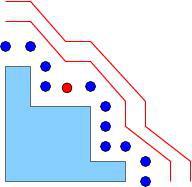borderbasix

Philippe Trebuchet & Bernard Mourrainborderbasix is a package for computing border basis and for solving zero-dimensional systems. Different types of coefficients can be used for the border basis computation: modular arithmetic, rational numbers, floating point numbers, ... The output is a monomial basis of the quotient algebra and the tables of multiplication by the variables in this basis, when the system is zero-dimensional. When the coefficients are rational or floating point numbers, the roots are computed over the complex numbers via eigenvalue/eigenvector computation.

Version: 0.1

Documentation: user manual, C++ manual